ASA Congruency Criteria

Congruence of Triangles
Concept wise

To prove two triangles congruent,

We use ASA Criteria –Angle Side Angle

In ASA Congruency Criteria,

• 2 angles of both the triangles are equal
• The side between these angles of both the triangles are equal.

For example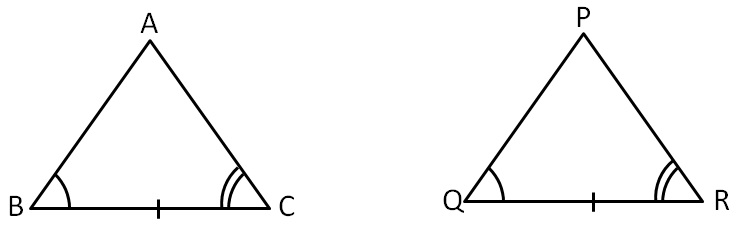Here,

2 angles and the side between them are equal.

So, they are congruent

Let’s take some examples

Are these triangles congruent?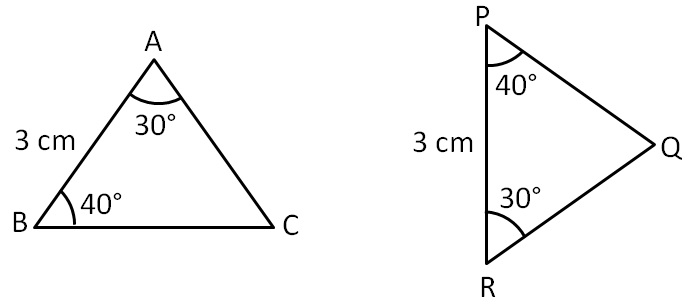In ∆ABC and ∆RPQ,

∠A = ∠R     ( Both are 30 °)

AB = RP     ( Both are 3 cm )

∠B  = ∠P    ( Both are 40 °)

∴ ∆ABC ≅ ∆RPQ    ( ASA Congruence Rule)

Here,

A R

B P

C Q

Are these triangles congruent?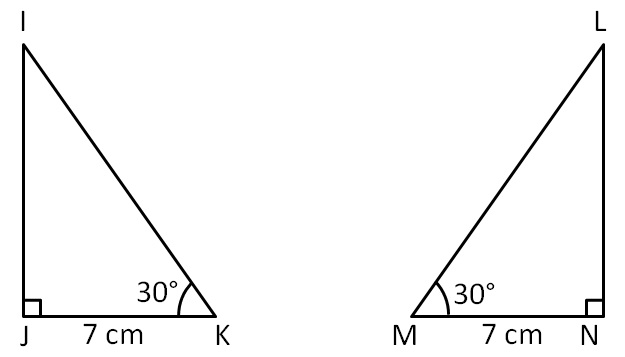In ∆IJK and ∆LNM,

∠J = ∠N      (Both are 90 °)

JK = NM     (Both are 7 cm )

∠K  = ∠M    (Both are 30 °)

∴ ∆IJK ≅ ∆LNM    (ASA Congruence Rule)

Here,

J N

K M

I L

Are these triangles congruent?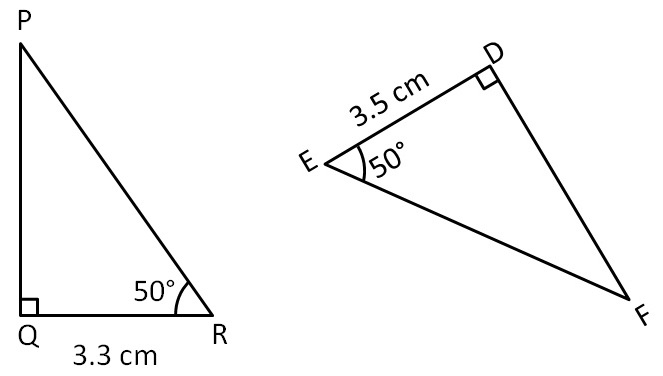In these two triangles,

the side is not same

So, these two triangles are not congruent

∴ ∆QRP ≇ ∆DEF

Are these triangles congruent?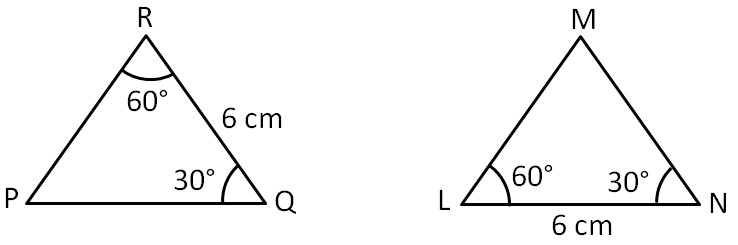In ∆PRQ and ∆MLN,

∠R = ∠L        ( Both are 60 °)

RQ = LN       ( Both  are 6 cm )

∠Q  = ∠N      (Both are 30 °)

∴ ∆PRQ ≅ ∆MLN    (ASA Congruence Rule)

Here,

R L

Q N

P M

Are these triangles congruent?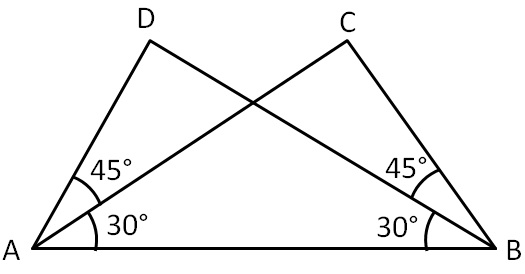= 30° + 45°

= 75°         …(1)

And, ∠ABC = ∠ABD + ∠CBD

= 30° + 45°

= 75°       …(2)

From (1) & (2)

In ∆ABD and ∆ BAC,

∠BAD = ∠ABC       (Proved above )

AB = BA         (Common )

∠DBA = ∠CAB     (Both are 30 °)

∴ ∆ABD ≅ ∆BAC      (ASA Congruency)

Here,

A B

B A

D C

#### Given below are measurements of some parts of two triangles. Examine whether the two triangles are congruent or not, by ASA congruence rule. In case of congruence, write it in symbolic form.

 ∆DEF ∆DEF (a) ∠D = 60°, ∠F = 80°, DF = 5 cm ∠Q = 60°, ∠R = 80°, QR = 5 cm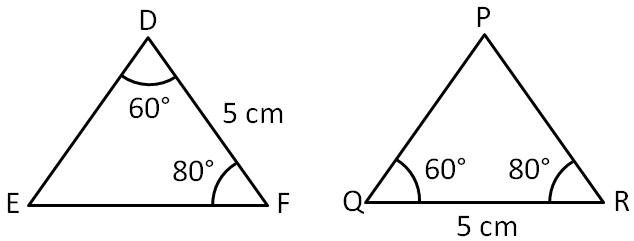In ∆DEF and ∆QPR,

∠D = ∠Q      (Both are 60 °)

DF = QP      (Both are 6 cm )

∠F = ∠R       (Both are 80 °)

∴ ∆DEF ≅ ∆QPR     (ASA Congruence Rule)

Here,

D Q

F R

E P

#### Given below are measurements of some parts of two triangles. Examine whether the two triangles are congruent or not, by ASA congruence rule. In case of congruence, write it in symbolic form.

 ∆DEF ∆DEF (a) ∠D = 60°, ∠F = 80°, DF = 6 cm ∠Q = 60°, ∠R = 80°, QP = 6 cm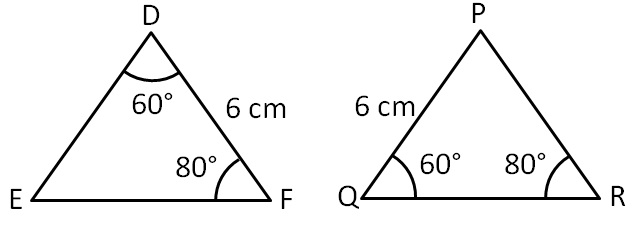In ∆DEF and ∆QPR,

∠D = ∠Q      (Both are 60 °)

DF = QR      (Both are 5 cm )

∠F = ∠R      (Both are 80 °)

∴ ∆DEF ≅ ∆QPR    (ASA Congruence Rule)

Here,

D Q

F R

E P

#### Given below are measurements of some parts of two triangles. Examine whether the two triangles are congruent or not, by ASA congruence rule. In case of congruence, write it in symbolic form.

 ∆DEF ∆DEF (a) ∠E = 80°, ∠F = 30°, EF = 5 cm ∠P = 80°, PQ = 5 cm, ∠R = 30° cm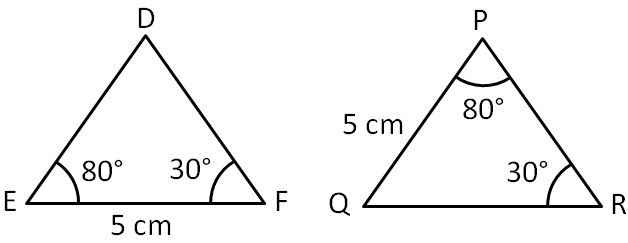Since PQ is not the side between ∠P and ∠R

Thus, side between two angles is not equal

∴ The triangles are not congruent

So, ∆DEF ≇ ∆QPR

#### State the three pairs of equal parts in triangles BAC and DAC.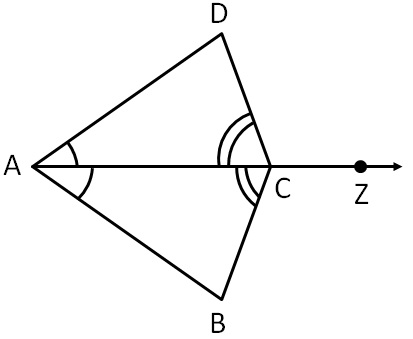In ∆BAC and ∆DAC,

∠BAC = ∠DAC      (AZ bisects ∠DAB )

AC = AC                 (Common )

∠BCA = ∠DCA     (AZ bisects ∠DCB )

∴  ∆BAC ≅ ∆DAC      (ASA Congruence Rule)

Learn in your speed, with individual attention - Teachoo Maths 1-on-1 Class